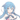# 算法复杂度分析(1)

2019-08-28折腾记录算法

## 大 O 复杂度表示法

_7int fun(int n) {_7 int num = 0;_7 for (int i = 1; i <= n; i++) {_7 sum = sum + i;_7 }_7 return sum;_7}

T(n) = O[f(n)]

## 时间复杂度分析

### 1. 只关注循环次数最多的代码

_7int fun(int n) {_7 int num = 0;_7 for (int i = 1; i <= n; i++) {_7 sum = sum + i;_7 }_7 return sum;_7}

### 2. 加法法则：只关注执行次数最多的代码的时间复杂度

_14int fun(int n) {_14 for (int i = 0; i < 10; i++) {_14 System.out.println("a");_14 }_14 for (int i = 0; i < n; i++) {_14 System.out.println("a");_14 }_14 for (int i = 0; i < n*10; i++) {_14 System.out.println("a");_14 }_14 for (int i = 0; i < n*n; i++) {_14 System.out.println("a");_14 }_14}

### 3. 乘法法则：嵌套代码的时间复杂度等于嵌套内外的时间复杂度的乘积

_11int fun(int n) {_11 for (int i = 0; i < n; i++) {_11 cal(n);_11 }_11}_11_11int cal(int n) {_11 for (int i = 0; i < n; i++) {_11 System.out.println("a");_11 }_11}

### 番外：常见的时间复杂度

#### 3. O(m+n)、O(m*n)

_8int fun(int m, int n) {_8 for (int i = 0; i < m; i++) {_8 System.out.println("a");_8 }_8 for (int i = 0; i < n; i++) {_8 System.out.println("a");_8 }_8}

## 空间复杂度分析

_4int fun(int n) {_4 int[] arr = new int[n];_4 return arr;_4}

## 结语

https://blog.ixk.me/post/algorithm-complexity-analysis-1
• 许可协议

BY-NC-SA

• 发布于

2019-08-28

• 本文作者

Otstar Lin

MySQL事务隔离一年来的经验总结